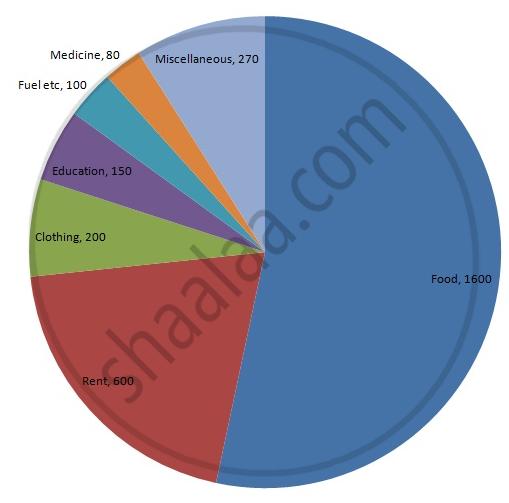Share

# Following is the Break up of the Expenditure of a Family on Different Items of Consumption: - Mathematics

Course
ConceptCircle Graph Or Pie Chart - Drawing Pie Charts

#### Question

Following is the break up of the expenditure of a family on different items of consumption:

 Items Food Clothing Rent Education Fuel etc. Medicine Miscellaneous Expenditure (in Rs) 1600 200 600 150 100 80 270

Draw a pie-diagram to represent the above data.

#### Solution

We know:
Central angle of a component = (component value/sum of component values x 360)
Here, total expenditure = Rs 3000
Thus, central angle for each component can be calculated as follows:

 Item Expenditure (in Rs) Sector angle Food 1600 1600/3000 x 360 = 192 Clothing 200 200/3000 x 360 = 24 Rent 600 600/3000 x 360 = 72 Education 150 150/3000 x 360 = 18 Fuel etc 100 100/3000 x 360 = 12 Medicine 80 80/3000 x 360 = 9.6 Miscellaneous 270 270/3000 x 360 = 32.4

Total : 3000 (in Rs)

Now, the pie chat representing the given data can be constructed by following the steps below:
Step 1 : Draw a circle of an appropriate radius.
Step 2 : Draw a vertical radius of the circle drawn in step 1.
Step 3 : Choose the largest central angle. Here, the largest central angle is 192o. Draw a sector with the central angle 192o in such a way that one of its radii coincides with the radius drawn in step 2 and another radius is in its counter clockwise direction.
Step 4 : Construct other sectors representing the other items in the clockwise sense in descending order of magnitudes of their central angles.
​Step 5 : Shade the sectors with different colours and label them as shown in the figure below.​​Is there an error in this question or solution?

#### APPEARS IN

RD Sharma Solution for Mathematics for Class 8 by R D Sharma (2019-2020 Session) (2017 to Current)
Chapter 25: Data Handling-III (Pictorial Representation of Data as Pie Charts or Circle Graphs)
Ex. 25.1 | Q: 16 | Page no. 14

#### Video TutorialsVIEW ALL 

Solution Following is the Break up of the Expenditure of a Family on Different Items of Consumption: Concept: Circle Graph Or Pie Chart - Drawing Pie Charts.
S